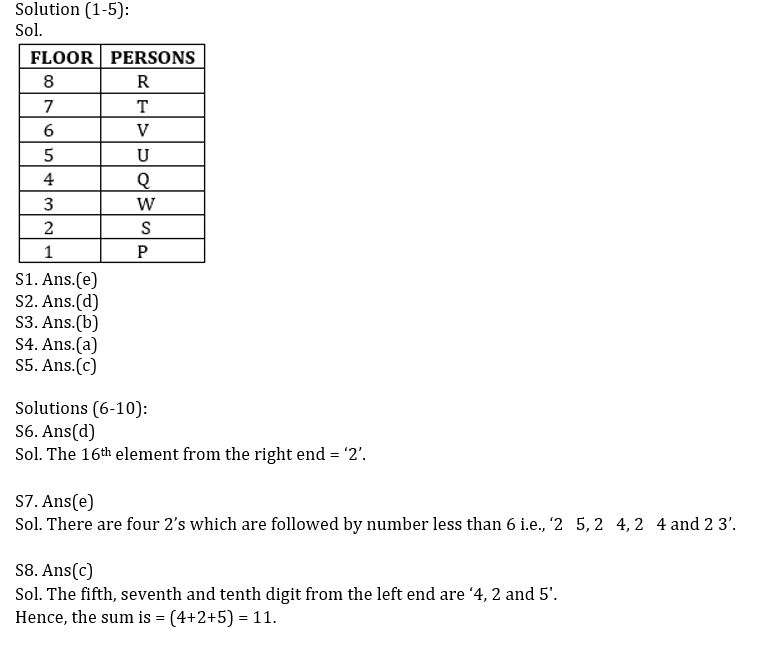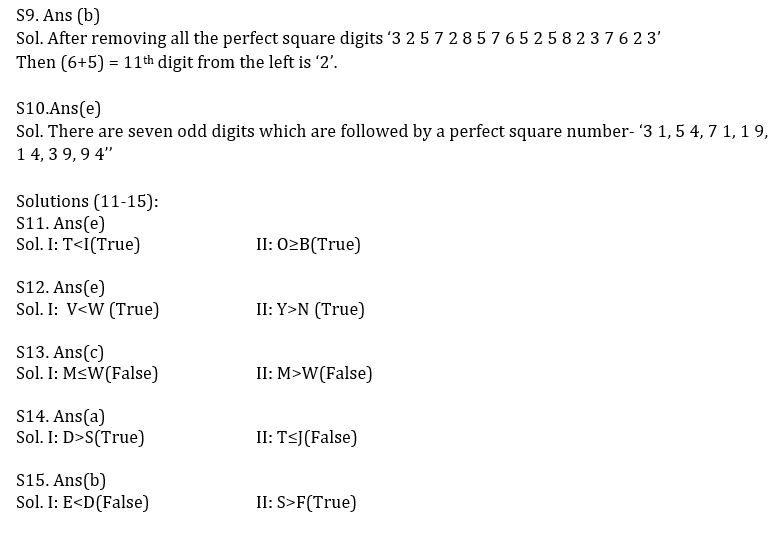Latest Banking jobs   »   Reasoning Ability Quiz For SBI Clerk...

# Reasoning Ability Quiz For SBI Clerk Prelims 2022- 8th May

Directions (1-5): Study the following information carefully and answer the questions given below-
Eight persons P, Q, R, S, T, U, V and W live on a separate floor each of an eight-floor building but not necessarily in the same order. The ground floor is numbered 1 and so on until the topmost floor is numbered 8.
U lives in an odd numbered floor. Four persons live between T and S who lives one of the floors below the floor on which U lives but not immediate below. R lives on the floor numbered which is multiple of 2 but not on 6th floor. V lives on an even numbered floor. More than two persons live between V and S. Q lives immediate above the floor on which W lives. R does not live below U. P lives on an odd numbered floor below T.

Attempt in Hindi

Q1. Who lives on the floor just above V?
(a) U
(b) P
(c) No one
(d) R
(e) T

Q2. How many persons live between S and V?
(a) None
(b) One
(c) Five
(d) Three
(e) Four

Q3. Which of the following pairs live on the first floor and the topmost floor respectively?
(a) R, Q
(b) P, R
(c) U, V
(d) S, P
(e) V, R

Q4. Who among the following lives on the fourth floor?
(a) Q
(b) S
(c) P
(d) T
(e) V

Q5. Which of the following combination is true?
(a) First floor-S
(b) Fourth floor-T
(c) Third floor-W
(d) Sixth floor- R
(e) Second floor- P

Directions (6-10): Study the following arrangement carefully and answer the questions given below:
3 1 2 5 4 7 2 8 4 5 7 1 9 6 5 2 4 1 4 5 8 2 4 3 9 7 6 9 4 2 3

Q6. Which of the following digit will be sixth to the left of tenth from the right end?
(a) 6
(b) 9
(c) 5
(d) 2
(e) None of these

Q7. How many 2’s is there in the given series which are followed by a number less than 6?
(a) One
(b) None
(c) Three
(d) Two
(e) More than three

Q8. What will be the addition of fifth, seventh and tenth digit from the left end?
(a) 13
(b) 10
(c) 11
(d) 15
(e) None of these

Q9. If all the perfect square digits are removed from the given series, then which of the following will be fifth to the right of sixth from the left end?
(a) 5
(b) 2
(c) 8
(d) 1
(e) None of these

Q10. How many odd numbers are there in the given series which are immediately followed by a perfect square digit?
(a) One
(b) None
(c) Three
(d) Five
(e) Seven

Directions (11-15): In these questions, relationship between different elements is show in the statements. The statements are followed by conclusions. Study the conclusions based on the given statements and select the appropriate answer:
(a) If only conclusion I follows.
(b) If only conclusion II follows.
(c) If either conclusion I or II follows
(d) If neither conclusion I nor II follows.
(e) If both conclusions I and II follow.

Q11. Statements: H>B=W≤I=O>P≥K=T
Conclusion I: T<I II: O≥B

Q12. Statements: R>E<W>P=Y≥V>C≥N
Conclusion I: V<W II: Y>N

Q13. Statements: T>R≥W=E≤N>C=D≤M
Conclusion I: M≤W II: M>W

Q14. Statements: J≥U≥Y>T≤D=V>H>S<K
Conclusion I: D>S II: T≤J

Q15. Statements: E=R <P≤S >D=C>I≥F
Conclusion I: E<D II: S>F

Solutions#### Congratulations!Union Budget 2023-24: Free PDF